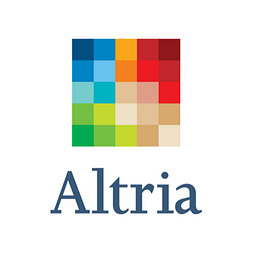# The Best Yielding Large Cap Consumer Stocks

+18.54%
Upside
43.97
Market
52.12
TrefisMO
Altria Group

Submitted by Dividend Yield as part of our contributors program.

Large Capitalized Consumer Goods Dividend Stocks With Highest Yield Researched By Dividend Yield – Stock, Capital, Investment. It’s very important to know what yields are traded within a sector or industry in order to compare risks and premiums. I made a screen of the best yielding large capitalized consumer goods stocks. 54 companies from the sector have a market capitalization over USD 10 billion and 49 of them pay dividends. As result, 20 stocks with a yield over 3 percent remained.

Below the results are three high yields of which Reynolds American (RAI) is the highest yielding stock, followed by Philips Electronics (PHG) and Altria (MO). The cigarettes industry has five representatives within the best yielding consumer stocks and covers the top dividend positions.

Relevant Articles

Here are my favorite stocks:

Altria Group (NYSE:MO) has a market capitalization of \$64.69 billion. The company employs 9,900 people, generates revenues of \$23,800.00 million and has a net income of \$3,393.00 million. The firm’s earnings before interest, taxes, depreciation and amortization (EBITDA) amounts to \$6,321.00 million. Because of these figures, the EBITDA margin is 26.56 percent (operating margin 25.50 percent and the net profit margin finally 14.26 percent).

Financial Analysis: The total debt representing 37.04 percent of the company’s assets and the total debt in relation to the equity amounts to 371.98 percent. Due to the financial situation, a return on equity of 76.13 percent was realized. Twelve trailing months earnings per share reached a value of \$1.67. Last fiscal year, the company paid \$1.58 in form of dividends to shareholders.

Market Valuation: Here are the price ratios of the company: The P/E ratio is 19.07, P/S ratio 2.72 and P/B ratio 17.66. Dividend Yield: 5.16 percent. The beta ratio is 0.41.

The Procter & Gamble (NYSE:PG) has a market capitalization of \$174.49 billion. The company employs 129,000 people, generates revenues of \$82,559.00 million and has a net income of \$11,797.00 million. The firm’s earnings before interest, taxes, depreciation and amortization (EBITDA) amounts to \$18,656.00 million. Because of these figures, the EBITDA margin is 22.60 percent (operating margin 19.16 percent and the net profit margin finally 14.29 percent).

Financial Analysis: The total debt representing 23.14 percent of the company’s assets and the total debt in relation to the equity amounts to 47.33 percent. Due to the financial situation, a return on equity of 18.32 percent was realized. Twelve trailing months earnings per share reached a value of \$3.21. Last fiscal year, the company paid \$1.97 in form of dividends to shareholders.

Market Valuation: Here are the price ratios of the company: The P/E ratio is 19.84, P/S ratio 2.11 and P/B ratio 2.65. Dividend Yield: 3.53 percent. The beta ratio is 0.44.

H.J. Heinz Company (NYSE:HNZ) has a market capitalization of \$17.54 billion. The company employs 34,800 people, generates revenues of \$10,706.59 million and has a net income of \$1,005.95 million. The firm’s earnings before interest, taxes, depreciation and amortization (EBITDA) amounts to \$1,932.42 million. Because of these figures, the EBITDA margin is 18.05 percent (operating margin 15.39 percent and the net profit margin finally 9.40 percent).

Financial Analysis: The total debt representing 37.72 percent of the company’s assets and the total debt in relation to the equity amounts to 148.38 percent. Due to the financial situation, a return on equity of 39.51 percent was realized. Twelve trailing months earnings per share reached a value of \$3.00. Last fiscal year, the company paid \$1.80 in form of dividends to shareholders.

Market Valuation: Here are the price ratios of the company: The P/E ratio is 18.30, P/S ratio 1.64 and P/B ratio 5.67. Dividend Yield: 3.50 percent. The beta ratio is 0.54.

PepsiCo (NYSE:PEP) has a market capitalization of \$104.47 billion. The company employs 297,000 people, generates revenues of \$66,504.00 million and has a net income of \$6,462.00 million. The firm’s earnings before interest, taxes, depreciation and amortization (EBITDA) amounts to \$12,242.00 million. Because of these figures, the EBITDA margin is 18.41 percent (operating margin 14.48 percent and the net profit margin finally 9.72 percent).

Financial Analysis: The total debt representing 36.73 percent of the company’s assets and the total debt in relation to the equity amounts to 130.04 percent. Due to the financial situation, a return on equity of 30.66 percent was realized. Twelve trailing months earnings per share reached a value of \$4.03. Last fiscal year, the company paid \$2.02 in form of dividends to shareholders.

Market Valuation: Here are the price ratios of the company: The P/E ratio is 16.58, P/S ratio 1.57 and P/B ratio 5.05. Dividend Yield: 3.22 percent. The beta ratio is 0.49.

Take a closer look at the full table of the best yielding large capitalized consumer stocks. The average price to earnings ratio (P/E ratio) amounts to 16.74 and forward P/E ratio is 14.82. The dividend yield has a value of 3.87 percent. Price to book ratio is 5.14 and price to sales ratio 1.75. The operating margin amounts to 15.82 percent.